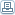# Simultaneous Despeckling & Compression of Med Ultrasound Images

 By: N.Karthikeyan and A.KanmaniA novel technique for despeckling the medical ultrasound images using lossy compression is presented. The logarithm of the input image is first transformed to the multiscale wavelet domain. It is then shown that the subband coefficients of the log transformed ultrasound image can be successfully modeled using the generalized Laplacian distribution. Based on this modeling, a simple adaptation of the zero-zone and reconstruction levels of the uniform threshold quantizer is proposed in order to achieve simultaneous despeckling and quantization. This adaptation is based on: 1) an estimate of the corrupting speckle noise level in the image; 2) the estimated statistics of the noise-free subband coefficients; and 3) the required compression rate. Context-based classification is also applied to the noisy coefficients to enhance the performance of the subband coder. The improvement that can be done in the existing is also discussed.STEP I : Perform the homomorphic operation by taking the logarithm of the image.STEP II : Decompose the corrupted image into detail levels using DWT which yields detail subband and an approximation subband.STEP III : Estimate the threshold level for the noise corrupted detail subband coefficients using BayesShrink.STEP IV:Perform the classification of the subband data into number of classes STEP V : Quantize each class using the data and rate adaptive quantizer The approximation subband is quantized using a uniform quantizer.STEP VI : Use an adaptive arithmetic coder to generate the encoded bit stream.
 Science